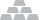Close

Documentation

Signature Calculation

Signature Version 2

Signature version 2 is an MD5 lowercase hash in the form of 32 digit hexadecimal number. Signature is calculated as follows:

sign = MD5("PARAM_NAME_1=PARAM_VALUE_1PARAM_NAME_2=PARAM_VALUE_2PARAM_NAME_3=PARAM_VALUE_3...SECRET_KEY")

Where SECRET_KEY is your project secret key. The additional parameters (e.g. PARAM_NAME_1=PARAM_VALUE_1PARAM_NAME_2=PARAM_VALUE_2) should be sorted alphabetically by the parameter name prior to hash calculation.

Signature Version 3

Signature version 3 is calculated the same way as version 2 but uses SHA256 hash instead of MD5.

sign = SHA256("PARAM_NAME_1=PARAM_VALUE_1PARAM_NAME_2=PARAM_VALUE_2PARAM_NAME_3=PARAM_VALUE_3...SECRET_KEY")

Where SECRET_KEY is your project secret key. The additional parameters (e.g. PARAM_NAME_1=PARAM_VALUE_1PARAM_NAME_2=PARAM_VALUE_2) should be sorted alphabetically by the parameter name prior to hash calculation.

Signature Version 1 (deprecated)

Signature version 1 is currenly supported for Digital Goods API and Virtual Currency API only. Please refer to the respective documentation pages for the details.

Signature Calculation Sample

Please note that Paymentwall API Libraries automatically calculate signatures for the Widget Call as well as for Pingback Validation.
If you still need to calculate the signature explicitly, below is an example of how this can be done using Paymentwall PHP Library at GitHub

Paymentwall_Config::getInstance()->set(array('private_key' => 'YOUR_PRIVATE_KEY'));
\$signature = (new Paymentwall_Signature_Widget())->calculate(
\$params, // widget params
\$signatureVersion // signature version
);

For other API libraries (Ruby, Python, Node.js, Java, .NET), signature can be calculated using Widget.calculateSignature method.